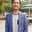Related Tags

d3
javascript

# How to create a pie chart using D3Shahpar Khan

D3 is an interactive JavaScript library for data visualization. It uses Scalar Vector Graphics (SVG) coupled with HTML and CSS to display charts and figures that illustrate the numeric data. You can also use D3 to make pie charts. Here is a step-by-step guide on how to make a pie chart using D3.

## Step 1: Dataset

Before even starting to code, we need a data set to base our chart on. For this example, we will take an array of objects where each object has two attributes: name and share.

var data = [{name: "Alex", share: 20.70},
{name: "Shelly", share: 30.92},
{name: "Clark", share: 15.42},
{name: "Matt", share: 13.65},
{name: "Jolene", share: 19.31}];


The shares sum up to 100!

## Step 2: D3 and SVG container

First, we need to import the D3 script using the src attribute and then initialize our SVG container with the appropriate width and height:

<script src="https://d3js.org/d3.v4.min.js"></script>
<svg width="500" height="400"></svg>


## Step 3: Set dimensions

We will make an svg variable and define its constraints keeping in mind the height and width we defined for our svg container in step 2. Then, we will make a radius variable. Ensure your radius is such that your pie chart does not exceed the svg container dimensions.

        var svg = d3.select("svg"),
width = svg.attr("width"),
height = svg.attr("height"),


## Step 4: Set scale

Since our data is ordinal, where we can divide data into categories (names) and order them (share), we will use scaleOrdinal. The domain will be our data set, and the range will be the colors associated with it.

        var ordScale = d3.scaleOrdinal()
.domain(data)
.range(['#ffd384','#94ebcd','#fbaccc','#d3e0ea','#fa7f72']);


## Step 5: Pie generator

Here, we first make a variable named pie that uses d3.pie(). The d3.pie() function takes in our numerical data (share) as values and uses it to generate valuable data to create a pie chart (start angle and end angle ). Using this pie variable, we will make the pie chart.

var pie = d3.pie().value(function(d) {
return d.share;
});

var arc = g.selectAll("arc")
.data(pie(data))
.enter();


## Step 6: Fill chart

We need to fill the pie chart with the colors we defined in our ordScale variable. First, we need to define the path, which will be the fill area for our chart. For that, we will define the outerRadius and innerRadius. After that, we will append this path to the arc variable we created in step 5 and fill it in using the ordScale.

        var path = d3.arc()

arc.append("path")
.attr("d", path)
.attr("fill", function(d) { return ordScale(d.data.name); });


Finally, we add the labels to our pie chart sections. Again, we need to define the area where we need to write the labels, create a label variable for that, and then supply the outerRadius and innerRadius to it. After that, we will append this text to the arc variable we created in step 5 and translate and style it accordingly.

        var label = d3.arc()

arc.append("text")
.attr("transform", function(d) {
return "translate(" + label.centroid(d) + ")";
})
.text(function(d) { return d.data.name; })
.style("font-family", "arial")
.style("font-size", 15);


Try changing innerRadius in line 44 from 0 to 50 to create a donut chart!

RELATED TAGS

d3
javascript

CONTRIBUTORShahpar Khan